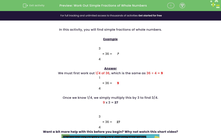# Work Out Simple Fractions of Whole Numbers

In this worksheet, students will find simple fractions of whole numbers by dividing and multiplying.Key stage:  KS 2

Curriculum topic:   Number: Fractions and Decimals

Curriculum subtopic:   Solve Fraction Problems

Difficulty level:#### Worksheet Overview

In this activity, we will be finding simple fractions of whole numbers.

Example

Find 3/4 of 36

We can write this as

3/4 x 36 = ?

We must first work out 1/4 of 36, which is the same as 36 ÷ 4 = 9

1/4 x 36 = 9

Once we know 1/4, we simply multiply this by 3 to find 3/4.

9 x 3 = 27

So, 3/4 of 36 = 27

At school you may have a little rhyme to help you to remember the order - here is one:

Divide the big number by the bottom number and times it by the top number!So, divide the big number (36) by the bottom number (4) and then times that answer (9) by the top number (3) - we get 27!!Want a bit more help with this before you begin? Why not watch this short video?

### What is EdPlace?

We're your National Curriculum aligned online education content provider helping each child succeed in English, maths and science from year 1 to GCSE. With an EdPlace account you’ll be able to track and measure progress, helping each child achieve their best. We build confidence and attainment by personalising each child’s learning at a level that suits them.

Get started